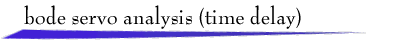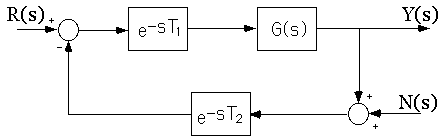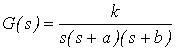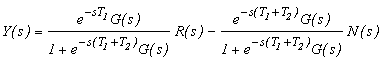A feedback system with a third-order plant transfer functionwhere both a and b are positive, and both forward-path and feedback-path time delays (transport lags) is driven by a reference input signal r(t) and a sensor noise signal n(t). Typical performance objectives involve tracking the reference signal while rejecting the influence of the sensor noise.

Time delays are commonly found in chemical process control systems, biological control systems, and control systems that involve long-distance communications. Typically, time delays have adverse effects on closed-loop stability properties.

The presence of time delays greatly complicates the analysis of control systems because the closed-loop transfer functionsare not rational functions of s. Therefore many standard techniques, such as the Routh-Hurwitz test for stability, and partial-fraction expansion methods for computing responses, do not apply. However the Nyquist stability criterion applies, and furthermore systems of the class considered here are stable for sufficiently low gain and become unstable as gain increases. Therefore Bode plots can be used to obtain meaningful values for phase margin and gain margin.

Shown below are the open-loop Bode magnitude and phase plots with the corner frequencies a and b marked on the magnitude plot. The gain and phase crossover frequencies are labeled. For this form of plant transfer function, it can be shown that closed-loop stability corresponds to positive phase margin. The left mouse button can be used to drag corner frequencies to desired locations, thereby adjusting the values of a, b, and k. The time delays are set by slider bars. The corresponding closed-loop Bode magnitude plot is also shown, with the closed-loop bandwidth labeled.

Two reference inputs can be selected, or click the Custom Input button and draw a reference input signal with the mouse. Also, high-frequency sensor noise can be selected at two amplitude levels. Plots of r(t) and y(t) are shown, and the Table button provides numerical values of important system parameters and response characteristics. (Rise time is the 10% - 90% rise time, and thus ignores an initial delay in the response. Mean-square steady-state error is computed on the range 15 < t < 25.)

The red-green lights show when computation is in process - it can take a bit of time on leisurely computers. Also note that the stability/instability border is imprecise: The instability sign is based on Phase Margin, though the numerical indication might be slightly different.

Exercises: Set the plant parameters to approximately k=100, a=5, b=10, and sketch the Custom Input r(t) = 2u(t) - 3u(t-1) + u(t-2), where u(t) is the unit step function.
1. What are the effects of T1 and T2 on Gain Margin and Phase Margin? Why are the effects the same?
2. What are the effects of T1 and T2 on closed-loop system bandwidth and resonant peak? Why are the effects the same?
3. What is the value of T1, with T2 = 0, that produces closed-loop instability?
4. What is the value of T2, with T1 = 0, that produces closed-loop instability?
5. Why should the answers to questions 3 and 4 be the same?
6. What is the effect of T1 on the time to peak amplitude of y(t)?
7. What is the effect of T2 on the time to peak amplitude of y(t)?
8. Why should the answers to questions 6 and 7 be different?return to demonstrations page

 Applet by Seth Kahn